Courses

# Test: Building With Bricks - 4

## 10 Questions MCQ Test Mathematics (Maths) for Class 4 | Test: Building With Bricks - 4

Description
This mock test of Test: Building With Bricks - 4 for Class 4 helps you for every Class 4 entrance exam. This contains 10 Multiple Choice Questions for Class 4 Test: Building With Bricks - 4 (mcq) to study with solutions a complete question bank. The solved questions answers in this Test: Building With Bricks - 4 quiz give you a good mix of easy questions and tough questions. Class 4 students definitely take this Test: Building With Bricks - 4 exercise for a better result in the exam. You can find other Test: Building With Bricks - 4 extra questions, long questions & short questions for Class 4 on EduRev as well by searching above.
QUESTION: 1

### Line m and four points are shown on the grid. Which two points appear to lie on the same line that is similar to line m?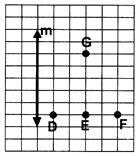Solution:

Points G and E appear to lie on the same line that is similar to line m.

QUESTION: 2

### The cube shown here was cut into three pieces- Which of the following groups CANNOT be the three pieces of the cube?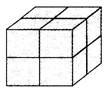Solution:

Among all the given options, option [C] cannot be the three pieces of the cube.

QUESTION: 3

### The perimeters of square P and rectangle Q are equal. Find the breadth of rectangle Q.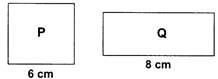Solution:

Perimeter of square P = 4 ×side = 4×6=24cm Perimeter of rectangle Q = 2 (Length + Breadth) = 2 (8 + Breadth) We are given Perimeter of P = Perimeter of Q ⇒24 = 2(8 + Breadth) ⇒24=16+2 ×Breadth ⇒2 × Breadth = 8 ⇒Breadth = 4 cm

QUESTION: 4

Veer plotted 3 points on a grid line. The three points together make a ______.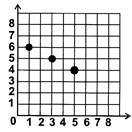Solution:

The three points will form a straight line.

QUESTION: 5

How many cubes are needed to make the given solid?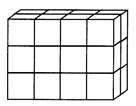Solution: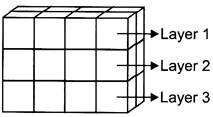Number of cubes in layer 1=8  Number of cubes in layer 2=8 Number of cubes in layer  3=8 Total number of cubes = 24

QUESTION: 6

Find the perimeter of the shaded part of the given Figure.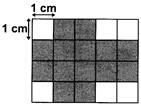Solution:

Counting the sides of the shaded squares while keeping in mind that common sides are not counted twice.

QUESTION: 7

Which of the following figures have a line of symmetry?

Solution:

Figure in option [D] have a line symmetry.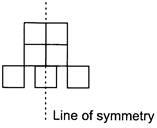QUESTION: 8

Study the given graph. Which of the given figures show pair of intersecting lines?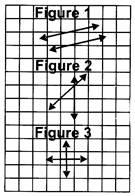Solution:

Two or more lines are said to be intersecting if they meet each other at a point. Here, Figure 2 and Figure 3 shows intersecting lines.

QUESTION: 9

Find the perimeter of the given figure.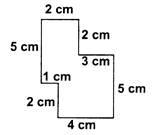Solution:

Perimeter of the given figure = 5 + 2 + 2 + 3 + 5 + 4 + 2 + 1 = 24cm

QUESTION: 10

Which of the following figures consists of exactly 6 faces?

Solution:

Figure in option [B] has exactly 6 faces.